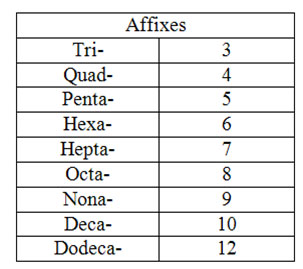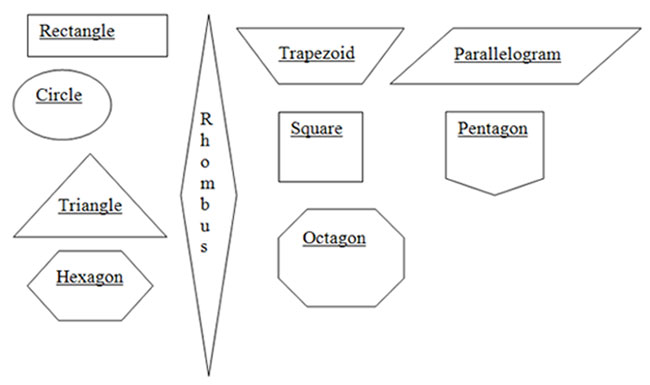Basic Geometric Shapes: Square, Circle, Rectangle, and Triangle

# Basic Geometric Shapes: Square, Circle, Rectangle, and Triangle

Before moving on to more advance mathematical concepts in algebra and geometry, it is first important to have a thorough understanding of geometric shapes. Everyone is familiar with the most common shapes: square, circle, rectangle, and triangle. Each of these shapes is created by combining specific amounts of lines and/or curves. A square, for example, is a four sided figure created by connecting four line segments. All of the line segments are of the same length and they come together to form four right angles.

A circle, on the other hand, has no straight lines. It is a combination of curves all connected. There are no angles to be found in a circle. A rectangle is created by connecting four line segments, in a similar manner to a square. However, a rectangle will typically have two line segments which are longer than the other two line segments. It could be described as an elongated square. Again, in a rectangle the four corners are created to form four right angles.

A triangle consists of three line segments connected. Unlike a square and a rectangle the angles in a triangle can be of various measurements and are not always right angles. Triangles often take their name from the type of angles which can be found within the triangle itself. A triangle which has one right angle can be referred to as a right triangle. An acute triangle is created when all of the angles within the triangle measure less than ninety degrees. An obtuse triangle is created when one angle within the triangle measures more than ninety degrees. Finally, an equiangular triangle is created when all of the angles within the triangle measure sixty degrees. Additionally, triangles can be labeled or identified by the type of sides they may have. A scalene triangle has no congruent sides. An isosceles triangle has two congruent sides. An equilateral triangle has three congruent sides. Equiangular and equilateral triangles are two different terms for the same triangle.

A polygon is a shape that is closed and is made up of only lines (no curves). It can have no open parts. Polygon in this case is a broader term to encompass a great many shapes including the square, rectangle and triangle. The circle described above cannot be considered a polygon because it is created by using curves, something which is not allowed by the simple definition of a polygon.

A parallelogram is named because the opposite sides of the shape are parallel. In order to determine if the sides can be considered parallel, one must examine them closely. Parallel lines will never intersect, or cross, no matter how long they are extended. Therefore in a shape, if one imagines the lines extended and extended through eternity and they never will meet or touch, then they can be considered parallel. However, if the lines meet or touch, they cannot be considered parallel and the shape could not then be considered a parallelogram. In this way, the shape of a triangle cannot be considered a parallelogram, as the two lines opposite of each other meet at the point of the triangle. Since the lines meet, they are not parallel.

An affix is prefix or a suffix which when added to a word changes the meaning of the word. Prefixes are added at the beginning of a word to change the meaning, while suffixes are added to the end of a word to change the meaning. Words can have a prefix, a suffix or both a prefix and a suffix. The broad term affix refers to both prefixes and suffixes. Typically, in geometric terms prefixes will be used frequently to help better define shapes. Prefixes themselves have specific meanings. It is these definitions which change the meanings of words. For example, the prefix un- means not. Therefore, if you add that prefix to the word able, which means capable of doing, the meaning is changed to mean not capable or not able. Prefixes are an important part in helping to determine the number of sides a specific polygon may have. For example, a pentagon uses the prefix pent- which means five because it has five sides. In the table below you will find some common prefixes which will help to distinguish how many sides a polygon will have.

Want to learn more? Take an online course in Geometry.Some other prefixes which you might find in geometry include:

• Circum- meaning around
• A or an – meaning not or without
• Ante- meaning before
• Anti – meaning against
• Con- meaning with or together
• Cycl- meaning circle or wheel
• Dia- meaning through, across or between
• Equi – meaning equal
• Exter- or Extra- meaning outside of
• Homo- meaning same
• Hyper- meaning above or over
• Hypo- meaning below or less than
• Morph- meaning shape
• Peri- meaning around
• Sect – meaning to cut
• Semi- meaning half
• Sub- meaning under or below
• Super- meaning above
• Trans- meaning across
• Vert- meaning to turn

This list is in no way totally complete, but understanding the meaning of basic prefixes can provide a more complete understanding of the terms, descriptions and definitions of items used throughout any study of geometry.

The following are examples of two-dimensional shapes you should be able to recognize:In addition to being able to recognize specific shapes, it is important to be able to classify shapes. When classifying shapes, one is looking for what common characteristics they share. Shapes can be classified and sorted in a variety of ways. Think about how you could sort the shapes listed above into two groups. The above shapes could be classified by the number of sides the have, those with curves and those with no curves, the number of angles and a variety of other ways. Some additional ways shapes can be classified include:

• Parallel lines – lines which if extended into infinity would never intersect. An example: railroad tracks
• Right angles – two lines which intersect and form a 90 degree angle. An example: extend your arm at the shoulder straight out and then bend your elbow so that your hand is raised to the ceiling the angle at your elbow is a 90 degree angle and thus a right angle.
• Curved lines/surfaces – any line/surface which is not straight. An example: a ball
• Two shapes that make another shape – An example: two squares put together make a new shape a rectangle
• Concentric circles - circles inside of circles
• Concave –shapes which have dents
• Convex – shapes without dents
• Shapes used over and over to make a pattern – An example: bricks
• Lines of Symmetry – a division of a shape where both parts look exactly the same – An example: square

The classification of shapes provides commonalities upon which further ideas and conclusions can be drawn. It is through this process of classification which becomes the basis for theorems or underlying principles. This allows for the better understanding of the world which surrounds man. For example, it is through the understanding of a variety of shapes that mosaic pictures or other tiled images can be created. Without a complete understanding of the similarities between shapes, a variety of uses would be too complicated to be completed.

It is through the understanding of these more simplistic properties or classifications that a deeper understanding can be based. For example, being able to recognize the idea of parallel lines within a group of shapes will allow for broader conclusions to be drawn. In this case, if shapes with parallel lines are combined longer, broader, and bigger parallel lines then exist. Therefore, combining shapes with parallel lines themselves create even more parallel lines. This can lead to a broader conclusion, combined parallel lines remain parallel. It is the ability to reach these broader conclusions that has lead to the determination of such ideas as theorems, which are then considered universal truths in the field of geometry.

Exercises:

• Using just the shapes above, how many different categories can you use to categorize them?
• Find an example of a shape in your home which matches each of the above identified categories.

Explore